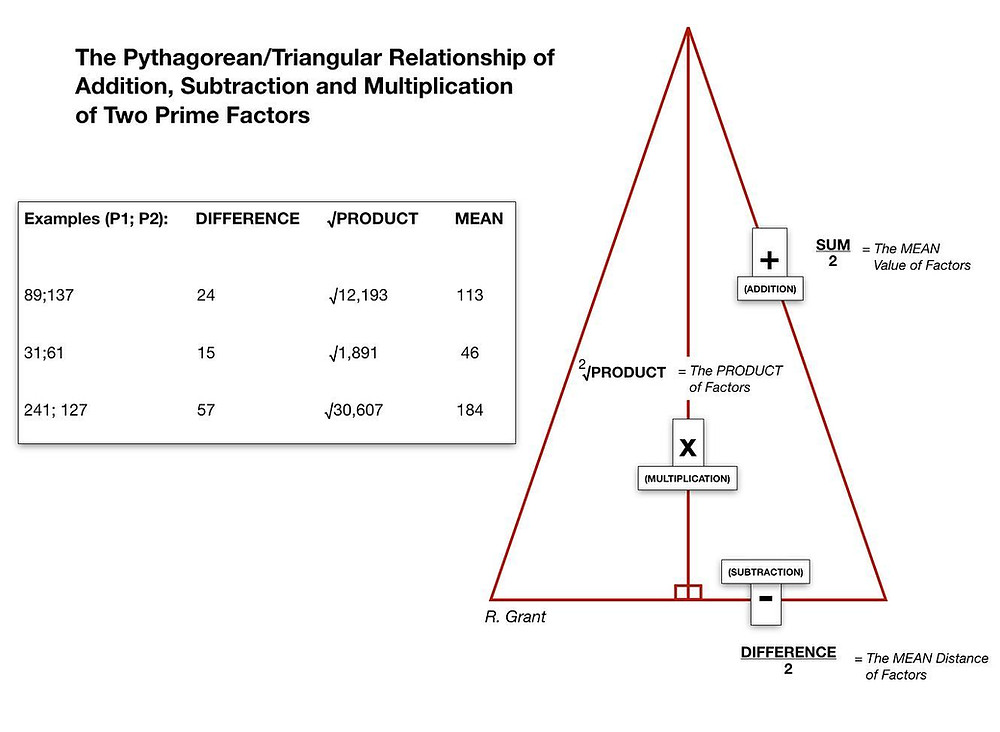Search

# The Pythagorean/Triangular Relationship of ADDITION, SUBTRACTION and MULTIPLICATION of Prime Factors

NEW MATHEMATICAL DISCOVERY (10/15/20): The Pythagorean/Triangular Relationship of ADDITION, SUBTRACTION and MULTIPLICATION of Prime Factors (Integers). Each pairing of integers is ENTANGLED through the sides of a Right Triangle. The Base side is determined as 1.) The SUBTRACTION (DIFFERENCE) of Two Prime Factors/2; 2.) The Height is the Square Root of the MULTIPLICATION (PRODUCT) of the same Two Prime Factors; and 3.) The Hypotenuse is determined by the ADDITION (SUM)/2 (MEAN of the same Two Prime Factors). As posited, this demonstrates the Entangled Relationship of the same Two Prime Integers' Product, Sum (Mean) and Difference (Mean) in forming the dimensions of a Right Triangle where ALL values are ONLY EVER WHOLE NUMBERS (swipe). An incredibly Elegant and Intelligent Design indeed.....Notice also the charge polarity from the equations themselves: POSITIVE (+), NEUTRAL (x), and NEGATIVE (-) ...perhaps pointing to a possible sub atomic relationship as well?